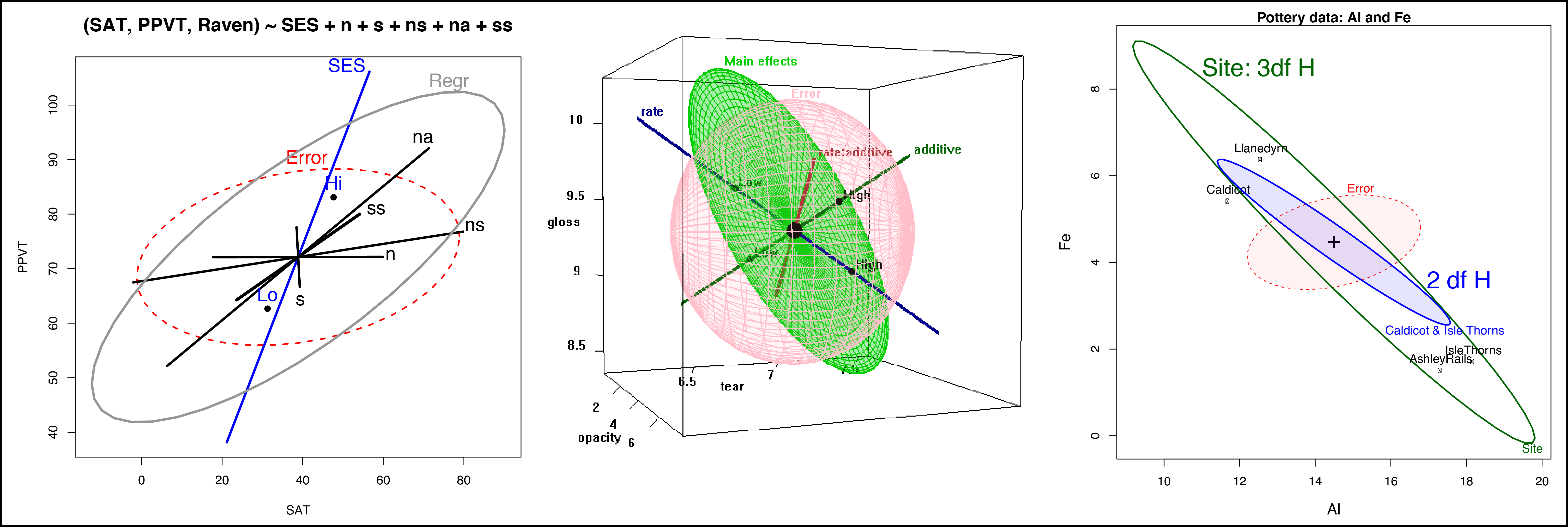# INTRODUCTION

This page aims to walk you through the procedures and analyses run during our presentation on “Recent Advances in Visualizing Multivariate Linear Models” at the 2013 Statistical Society of Canada annual conference. The following examples use three primary datasets: `iris` (a `base` dataset), and `Pottery` and `Rohwer`, both of which are included in the `heplots` package.

``````library(heplots)
``````
``````##   Sepal.Length Sepal.Width Petal.Length Petal.Width Species
## 1          5.1         3.5          1.4         0.2  setosa
## 2          4.9         3.0          1.4         0.2  setosa
## 3          4.7         3.2          1.3         0.2  setosa
## 4          4.6         3.1          1.5         0.2  setosa
## 5          5.0         3.6          1.4         0.2  setosa
## 6          5.4         3.9          1.7         0.4  setosa
``````
``````summary(iris)
``````
``````##   Sepal.Length   Sepal.Width    Petal.Length   Petal.Width        Species
##  Min.   :4.30   Min.   :2.00   Min.   :1.00   Min.   :0.1   setosa    :50
##  1st Qu.:5.10   1st Qu.:2.80   1st Qu.:1.60   1st Qu.:0.3   versicolor:50
##  Median :5.80   Median :3.00   Median :4.35   Median :1.3   virginica :50
##  Mean   :5.84   Mean   :3.06   Mean   :3.76   Mean   :1.2
##  3rd Qu.:6.40   3rd Qu.:3.30   3rd Qu.:5.10   3rd Qu.:1.8
##  Max.   :7.90   Max.   :4.40   Max.   :6.90   Max.   :2.5
``````
``````head(Pottery)
``````
``````##        Site   Al   Fe   Mg   Ca   Na
## 1 Llanedyrn 14.4 7.00 4.30 0.15 0.51
## 2 Llanedyrn 13.8 7.08 3.43 0.12 0.17
## 3 Llanedyrn 14.6 7.09 3.88 0.13 0.20
## 4 Llanedyrn 11.5 6.37 5.64 0.16 0.14
## 5 Llanedyrn 13.8 7.06 5.34 0.20 0.20
## 6 Llanedyrn 10.9 6.26 3.47 0.17 0.22
``````
``````summary(Pottery)
``````
``````##           Site          Al             Fe             Mg             Ca              Na
##  AshleyRails: 5   Min.   :10.1   Min.   :0.92   Min.   :0.53   Min.   :0.010   Min.   :0.030
##  Caldicot   : 2   1st Qu.:11.9   1st Qu.:1.70   1st Qu.:0.67   1st Qu.:0.060   1st Qu.:0.050
##  IsleThorns : 5   Median :13.8   Median :5.46   Median :3.83   Median :0.155   Median :0.150
##  Llanedyrn  :14   Mean   :14.5   Mean   :4.47   Mean   :3.14   Mean   :0.147   Mean   :0.159
##                   3rd Qu.:17.4   3rd Qu.:6.59   3rd Qu.:4.50   3rd Qu.:0.215   3rd Qu.:0.215
##                   Max.   :20.8   Max.   :7.09   Max.   :7.23   Max.   :0.310   Max.   :0.540
``````
``````head(Rohwer)
``````
``````##   group SES SAT PPVT Raven n  s ns na ss
## 1     1  Lo  49   48     8 1  2  6 12 16
## 2     1  Lo  47   76    13 5 14 14 30 27
## 3     1  Lo  11   40    13 0 10 21 16 16
## 4     1  Lo   9   52     9 0  2  5 17  8
## 5     1  Lo  69   63    15 2  7 11 26 17
## 6     1  Lo  35   82    14 2 15 21 34 25
``````
``````summary(Rohwer)
``````
``````##      group      SES          SAT            PPVT           Raven            n
##  Min.   :1.00   Hi:32   Min.   : 1.0   Min.   : 40.0   Min.   : 8.0   Min.   : 0.00
##  1st Qu.:1.00   Lo:37   1st Qu.:14.0   1st Qu.: 59.0   1st Qu.:12.0   1st Qu.: 1.00
##  Median :1.00           Median :35.0   Median : 71.0   Median :14.0   Median : 3.00
##  Mean   :1.46           Mean   :38.9   Mean   : 72.1   Mean   :14.1   Mean   : 3.49
##  3rd Qu.:2.00           3rd Qu.:58.0   3rd Qu.: 82.0   3rd Qu.:16.0   3rd Qu.: 5.00
##  Max.   :2.00           Max.   :99.0   Max.   :105.0   Max.   :21.0   Max.   :20.00
##        s               ns           na             ss
##  Min.   : 0.00   Min.   : 3   Min.   :11.0   Min.   : 8.0
##  1st Qu.: 4.00   1st Qu.:10   1st Qu.:18.0   1st Qu.:16.0
##  Median : 7.00   Median :14   Median :25.0   Median :21.0
##  Mean   : 7.07   Mean   :14   Mean   :23.3   Mean   :19.8
##  3rd Qu.:10.00   3rd Qu.:18   3rd Qu.:27.0   3rd Qu.:24.0
##  Max.   :18.00   Max.   :28   Max.   :35.0   Max.   :32.0
``````

The script is organized sequentially based upon the plots used in our presentation. However, plots shown during the background overview that are repeated in a later section are only shown in the pertinent subsection. The structure is as follows:

1. Background and Visual Overview
2. Hypothesis-Error (HE) Plots
• MANOVA Designs
• Multiple Regression Designs
3. Reduced-Rank Displays
• Canonical Disciminant HE Plots
4. Recent Extensions
• Canonical Correlation
• Robust Multivariate Models
• Influence Diagnostics for Multivariate Models

# BACKGROUND and VISUAL OVERVIEW

To contrast the novel developments in data visualization techniques, we begin by demonstrating some traditional techniques and how they are used to convey information about more simplistic designs.

Our first graphics demonstrate some of the traditional bivariate approaches to visualizing data. These figures are created using the `lattice` package, which allows the user to request a data concentration ellipse be superimposed on the plots.

``````library(lattice)
library(latticeExtra)

# A simple grouped scatterplot:
xyplot(Sepal.Length/10 ~ Petal.Length/10, group = Species, data = iris,
# Define axes:
xlab = "Petal length (mm)", ylab = "Sepal length (mm)",
# Define legend parameters:
auto.key = list(x = .1, y = .8, corner = c(0, 0)),
scales = "free", par.settings=list(superpose.symbol=list(pch=1:3)))
``````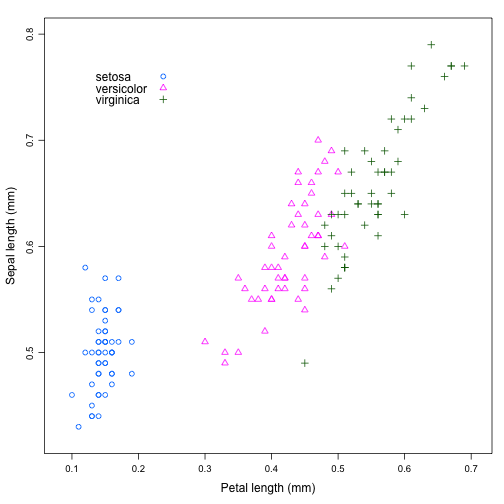``````# A grouped scatterplot with 95% data concentration ellipses:
xyplot(Sepal.Length/10 ~ Petal.Length/10, groups=Species, data = iris,
# Define axes:
xlab = "Petal length (mm)", ylab = "Sepal length (mm)",
# Define legend parameters:
auto.key = list(x = .1, y = .8, corner = c(0, 0)), scales = "free",
par.settings = list(superpose.symbol = list(pch=c(1:13)), superpose.line = list(lwd=2, lty=1:3)),
# Superimpose data ellipse on the scatterplot:
panel = function(x, y, ...) {
panel.xyplot(x, y, ...)
panel.ellipse(x, y, ...)
}
)
``````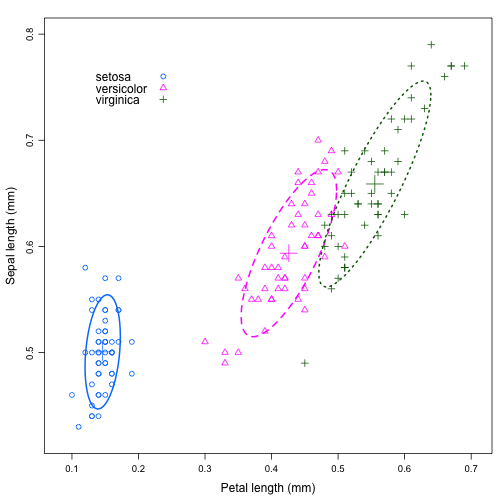With additional variables (the `iris` dataset contains four continuous variables that are of interest, and one categorical variable that is used for grouping), we can look at each of the bivariate relationships in turn. For instance, using the `car` package, we can produce a scatterplot matrix that is annotated to include data concentration ellipses for each group:

``````library(car)
scatterplotMatrix(~ Sepal.Length + Sepal.Width + Petal.Length + Petal.Width | Species,
data = iris, diagonal = "none", smoother = FALSE, reg.line = lm,
ellipse = TRUE, by.groups = TRUE)
``````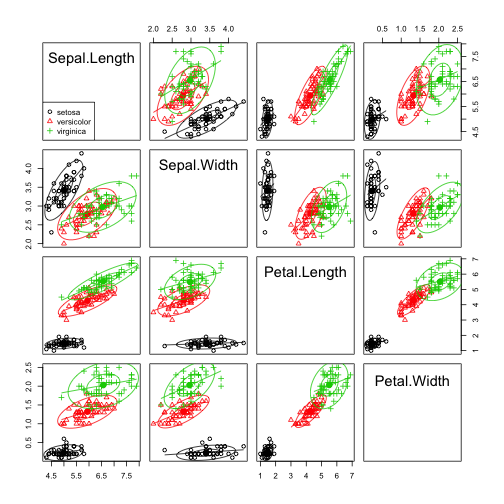Finally, utilizing a data reduction technique, we can look at a biplot, which demonstrates the relationship between the variables in terms of how each aids in accounting for the maximum amount of variation found in the data.

``````# A biplot showing the maximum total variation by species:
library(bpca)
library(car)
iris.pca <- bpca(iris[,-5], method = "sqrt", scale = TRUE)
scores <- iris.pca\$coord\$objects[,1:2]
col <- c('blue', 'darkgreen', 'red')[unclass(iris\$Species)]
plot(iris.pca,
var.factor = .24, var.cex = 1.2, var.col = "black", lwd = 2,
obj.names = FALSE, obj.cex = 1, obj.col = col,
obj.pch = (15:17)[unclass(iris\$Species)], xpd = TRUE)

dataEllipse(scores, groups=iris\$Species, add=TRUE, levels=0.68, col=unique(col), plot.points=FALSE)
``````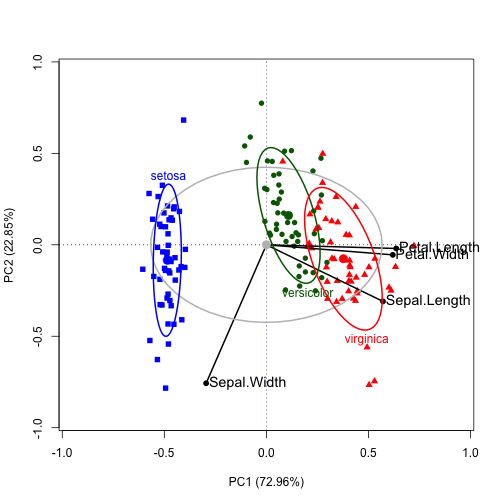This narrative follows our substantive example, using the Romano-British `Pottery` data, which has five continuous variables designating concentration of minerals in a particular piece and one categorical variable regarding the site at which it was found.

``````pottery.mod <- lm(cbind(Al, Fe, Mg, Ca, Na) ~ Site, data = Pottery)
pottery.man <- Manova(pottery.mod)
summary(pottery.man)
``````
``````##
## Type II MANOVA Tests:
##
## Sum of squares and products for error:
##         Al       Fe       Mg       Ca      Na
## Al 48.2881  7.08007  0.60801  0.10647 0.58896
## Fe  7.0801 10.95085  0.52706 -0.15519 0.06676
## Mg  0.6080  0.52706 15.42961  0.43538 0.02762
## Ca  0.1065 -0.15519  0.43538  0.05149 0.01008
## Na  0.5890  0.06676  0.02762  0.01008 0.19929
##
## ------------------------------------------
##
## Term: Site
##
## Sum of squares and products for the hypothesis:
##          Al       Fe       Mg      Ca      Na
## Al  175.610 -149.296 -130.810 -5.8892 -5.3723
## Fe -149.296  134.222  117.745  4.8218  5.3259
## Mg -130.810  117.745  103.351  4.2092  4.7105
## Ca   -5.889    4.822    4.209  0.2047  0.1548
## Na   -5.372    5.326    4.711  0.1548  0.2582
##
## Multivariate Tests: Site
##                  Df test stat approx F num Df den Df   Pr(>F)
## Pillai            3      1.55     4.30     15  60.00 2.41e-05 ***
## Wilks             3      0.01    13.09     15  50.09 1.84e-12 ***
## Hotelling-Lawley  3     35.44    39.38     15  50.00  < 2e-16 ***
## Roy               3     34.16   136.64      5  20.00 9.44e-15 ***
## ---
## Signif. codes:  0 '***' 0.001 '**' 0.01 '*' 0.05 '.' 0.1 ' ' 1
``````
``````op <- par(mfrow = c(2,3))
boxplot(Al ~ Site, data = Pottery, main = "Al")
boxplot(Fe ~ Site, data = Pottery, main = "Fe")
boxplot(Mg ~ Site, data = Pottery, main = "Mg")
boxplot(Ca ~ Site, data = Pottery, main = "Ca")
boxplot(Na ~ Site, data = Pottery, main = "Na")
par(op)
``````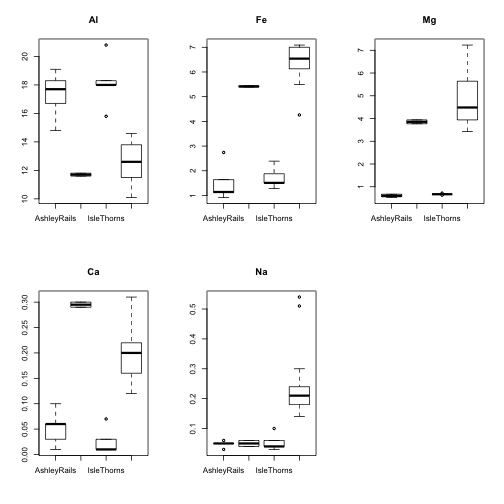# HYPOTHESIS-ERROR PLOTS

## MANOVA Designs

The univariate displays are limited - they show the variation in the elements at the different sites, but they do not show which elements group together. A more novel approach for working with a multivariate linear models with two dependent variables is to use a Hypothesis-Error (HE) plot. In this plot, we take the regression of petal length and sepal length on species from the `iris` dataset.

``````iris.mod <- lm(cbind(Petal.Length, Sepal.Length) ~ Species, data = iris)
heplot(iris.mod, xlab = "Petal Length in cm.", ylab = "Sepal length in cm.", size = "effect")
``````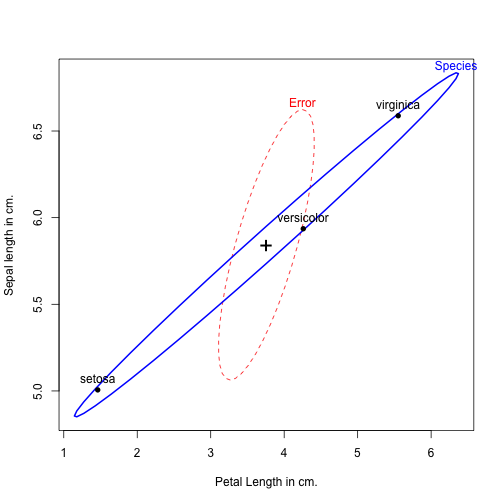Similarly, with the `Pottery` data, we can look at 2-D HE plots to show how the sites differ on two elements at a time. These plots can also be configured to display different scaling coefficients for the plotting of the hypothesis ellipse relative to the error ellipse.

``````# Obtain limits from the model:
pot <- heplot(pottery.mod)
``````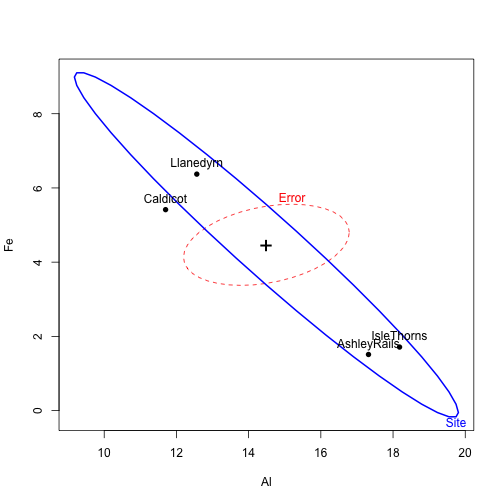``````# 2-D HE Plot for Aluminum and Iron with "Effect" Scaling (with annotations):
heplot(pottery.mod, size="effect.size",
xlim = pot\$xlim, ylim = pot\$ylim, main = "Pottery data: Al and Fe",
fill = c(TRUE, TRUE), fill.alpha = 0.05, cex.lab = 1.25)
label <- expression(paste("Effect scaling:", H / df[e]))
text(12.6, 7.2, label, col = "blue", cex = 1.5, pos = 4)
label <- expression( E / df[e])
text(16.8, 5.2, label, col = "red", cex = 1.5, pos = 4)

# Addition of the ellipse generated with "Significance" Scaling:
heplot(pottery.mod, add = TRUE, col = "darkgreen", fill = c(FALSE, TRUE), fill.alpha = 0.1)
label <- expression(paste("Significance scaling: H / ",  lambda[alpha], df[e]))
text(10.6, 8.7, label, col = "darkgreen", cex = 1.5, pos = 4)
``````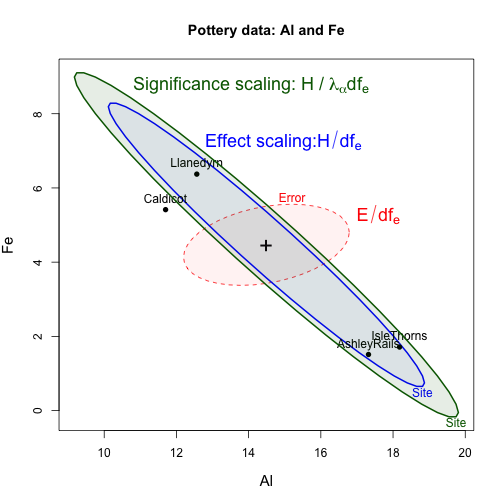The HE plot is also amenable to visualizing particular contrasts and linear hypotheses.

``````# 2-D HE plot for Aluminum and Iron with 3 degree of freedom hypothesis:
heplot(pottery.mod, col = c("red", "darkgreen"), main = "Pottery data: Al and Fe",
fill = c(TRUE,FALSE), fill.alpha = 0.05, cex.lab = 1.25)
text(12.5, 8.5, "Site: 3df H", col = "darkgreen", cex = 2)
``````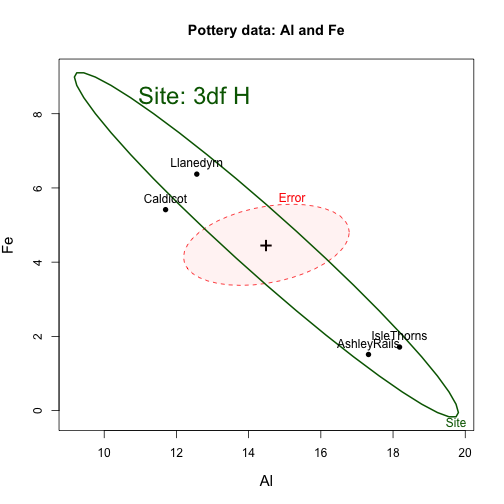``````heplot(pottery.mod, col = c("red", "darkgreen"), main = "Pottery data: Al and Fe",
fill = c(TRUE,FALSE), fill.alpha = 0.05, cex.lab = 1.25)
text(12.5, 8.5, "Site: 3df H", col = "darkgreen", cex = 2)
# Addition of a 2 degree of freedom hypothesis:
heplot(pottery.mod, terms = FALSE, add = TRUE, col = "blue",
hypotheses = list("Caldicot & Isle Thorns" = c("SiteCaldicot = 0", "SiteIsleThorns = 0")),
fill = c(FALSE, TRUE), fill.alpha = 0.1)
text(17.8, 3.6, "2 df H", col="blue", cex=2)
``````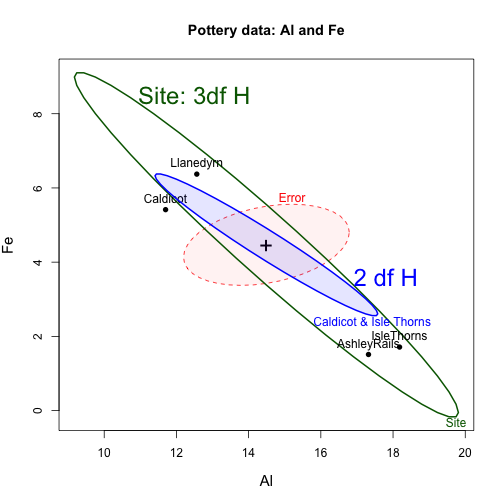``````heplot(pottery.mod, col = c("red", "darkgreen"), main = "Pottery data: Al and Fe",
fill = c(TRUE,FALSE), fill.alpha = 0.05, cex.lab = 1.25)
text(12.5, 8.5, "Site: 3df H", col = "darkgreen", cex = 2)
# Addition of a 2 degree of freedom hypothesis:
heplot(pottery.mod, terms = FALSE, add = TRUE, col = "blue",
hypotheses = list("Caldicot & Isle Thorns" = c("SiteCaldicot = 0", "SiteIsleThorns = 0")),
fill = c(FALSE, TRUE), fill.alpha = 0.1)
text(17.8, 3.6, "2 df H", col="blue", cex=2)
# Addition of a 1 degree of freedom hypothesis:
heplot(pottery.mod, terms = FALSE, add = TRUE, col="brown",
hypotheses=list("C-A" = "SiteCaldicot", "I-A" = "SiteIsleThorns"))
text(13.1, 4.5, "1 df H", col="brown", cex=1.7)
``````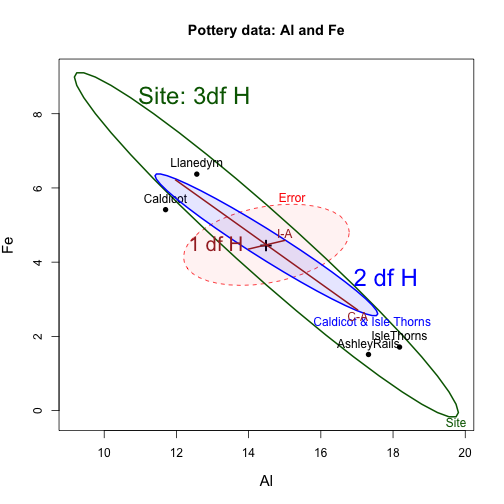With more than two response variables, we can also visualize all pairs using a generalization of the scatterplot matrix:

``````# With the iris dataset:
iris.mod <- lm(cbind(Sepal.Length, Sepal.Width, Petal.Length, Petal.Width) ~ Species, data = iris)
pairs(iris.mod)
``````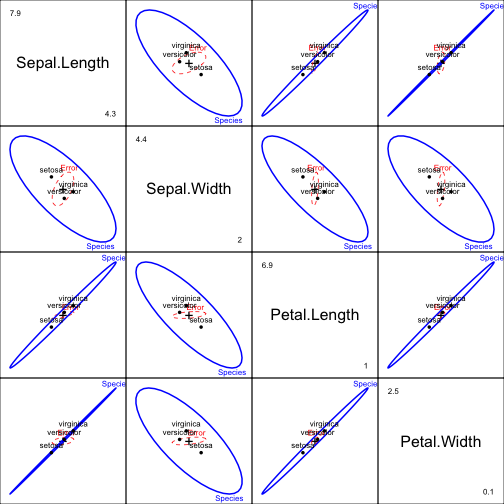``````# With the Pottery dataset:
pairs(pottery.mod, fill=TRUE, fill.alpha=0.1)
``````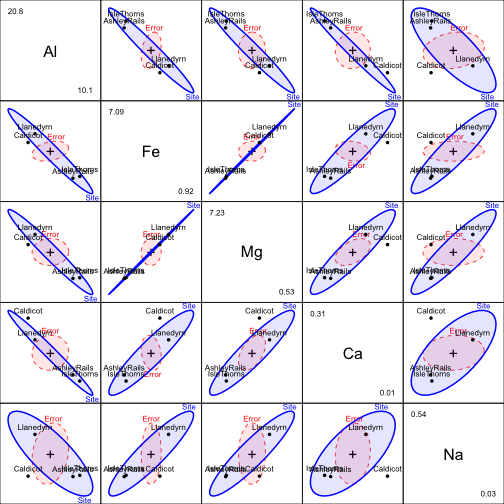Finally, we can also produce an interactive visualization that yields information on how three variables behave simultaneously with `heplot3d()`:

``````# Uncomment the heplot3d line to produce the plot.
# It can be rotated to view the data cloud from all sides.
# Note: This visualization will load in a new window.
# heplot3d(pottery.mod)
``````

Unfortunately, the 3D plot can only show three variables at a time, and as such cannot express all of the relationships at play.

## Multivariate Multiple Regression

HE plots are also useful in multivariate multiple regression, where the predictor variables are quantitative. In this design, we often test two primary sets of hypotheses: one, to see is all coefficients for each of the responses is zero (overall test); and two, for the significance of the individual predictor slopes.

This process can be demonstrated using Rohwer's data on paired-associate (PA) tasks, the five continuous predictors, and how they measure intelligence and achievement, which was captured using three scales, the SAT, the Peabody Picture Vocabulary Test, and the Raven Progressive Matrices test.

``````# Multivariate regression model for low SES students:
rohwer.ses2 <- lm(cbind(SAT, PPVT, Raven) ~ n + s + ns + na + ss, data = Rohwer, subset = SES=="Lo")

# Test of infidivudal predicators (lines) and overall test (blue ellipse):
he <- heplot(rohwer.ses2, col=c("red", rep("black", 5), "blue"),
hypotheses = list("Overall: B = 0" = c("n", "s", "ns", "na", "ss")),
cex = 1.25, fill = c(TRUE, TRUE), fill.alpha = c(.05, .1),
xlab = "Student Achievement Test",
ylab = "Peabody Picture Vocabulary Test",
cex.lab = 1.25, xlim = c(-10, 70), ylim = c(30, 90))
``````